# End-to-End Speech Recognition: Part 1 – Neural Networks for Executives (I Mean Dummies)When I originally contemplated the subject of my next blog post, I thought it might be interesting to provide a thorough explanation of the latest and greatest speech recognition algorithms, often referred to as End-to-End Speech Recognition, Deep Speech, or Connectionist Temporal Classification (CTC).   However, as I began to research the topic I quickly discovered that my basic knowledge of neural networks was woefully lacking.  Several weeks of reading and a few hundred lines of code later, I realized before I could teach a fellow plebe like myself about end-to-end speech recognition,  I probably needed to introduce the fundamentals first.

With that in mind, what was intended to be a single entry will likely turn into multiple blog posts covering an overview of end-to-end speech recognition and some fundamentals of deep learning that make it possible.  In this first post I’d like to provide a brief introduction to end-to-end speech recognition and then give a more detailed tutorial about one of the basic components of deep learning, a multilayer perceptron, also known as a feed forward neural network.  I’ll then walk you through how I brought all this information together while building a very basic end-to-end speech recognition system.

##### End-to-End Speech Recognition

So what is end-to-end speech recognition anyway?  At it’s most basic level an end-to-end speech recognition solution aims to train a machine to convert speech to text by directly piping raw audio input with associated labeled text through a deep learning algorithm.   The resulting model is then able to recognize speech with no further algorithmic components.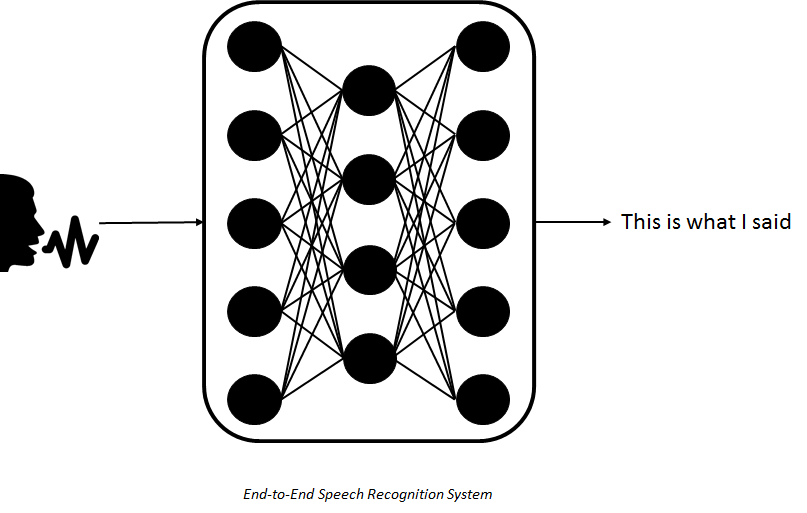And why is this any better than traditional speech recognition systems?  Traditional speech recognition systems use a much more complicated architecture that includes feature generation, acoustic modeling, language modeling, and a variety of other algorithmic techniques in order to be accurate and effective.   This in turn makes the training, testing, and code complexity far more difficult than would be with an end-to-end system.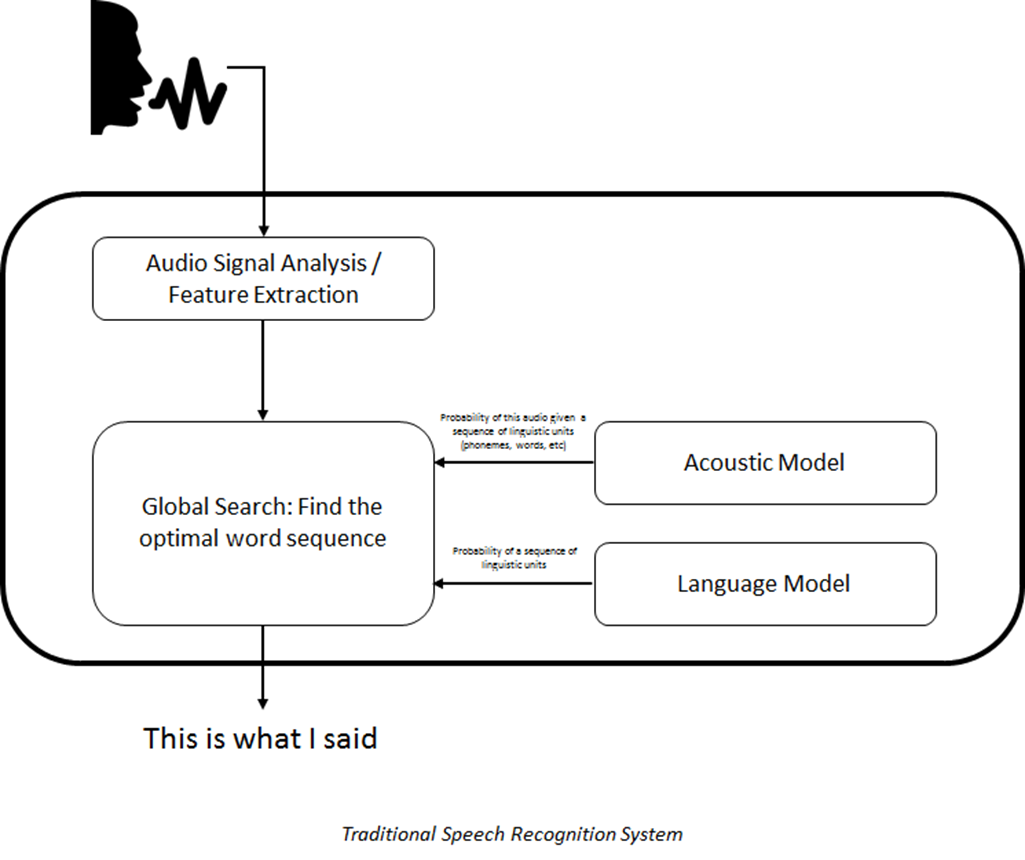In other words an end-to-end solution greatly reduces the complexity in building a speech recognition system.   And if that alone doesn’t convince you of the value an end-to-end recognizer brings to the table, several research teams, most notably the folks at Baidu, have shown that they can achieve superior accuracy results over traditional speech recognition systems.

To validate the possibilities of an end-to-end speech recognition system I decided to build my own.  However, I quickly found that building such a system required advanced knowledge of deep learning techniques.   This is because the current end-to-end systems generally rely on more complex neural network algorithms like Recurrent Neural Networks (RNNs) and something called the connectionist temporal loss function that are difficult to understand if you don’t have a solid understanding of basic neural networks.   So I opted to take a simpler approach and see if I could build a very simple end-to-end recognizer using basic deep learning techniques.   Specifically a feed forward neural network or multi layer perceptron.

##### Neural Network Fundamentals

Before I dive into the details, let me provide a quick tutorial on the feed forward neural network.  The underlying element of a neural network is called a perceptron or an artificial neuron.  Much like a biological neuron, a perceptron takes a series of inputs, performs a function on those inputs, and produces and output that can be passed to other neurons.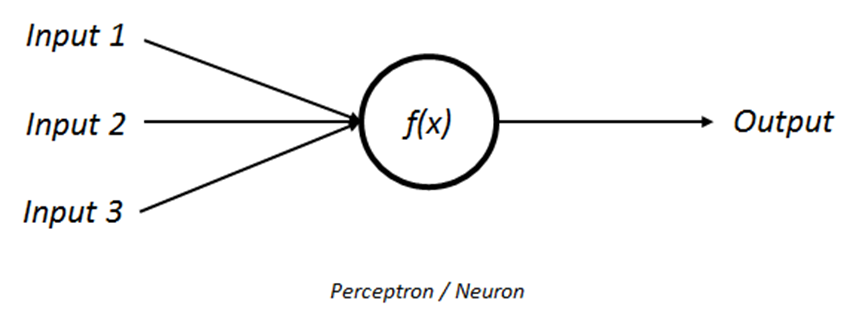The simplest function is just a sum of weighted inputs.  However this function is a linear relationship and the world is rarely linear so we apply something called an activation function to help impart nonlinearity.   There are actually numerous activation functions used in neural networks, some linear and some not, but the Sigmoid and TanH functions are two you will commonly see in the relevant literature.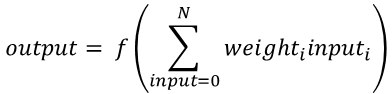Now that we know what a neuron is, a neural network is really just a collection of multiple interconnected neurons.   Neurons are grouped and connected in “layers”.   The simplest neural network is a single layer network that connects one or more inputs to one or more outputs.   There is no calculation on the input layer, only the output layer.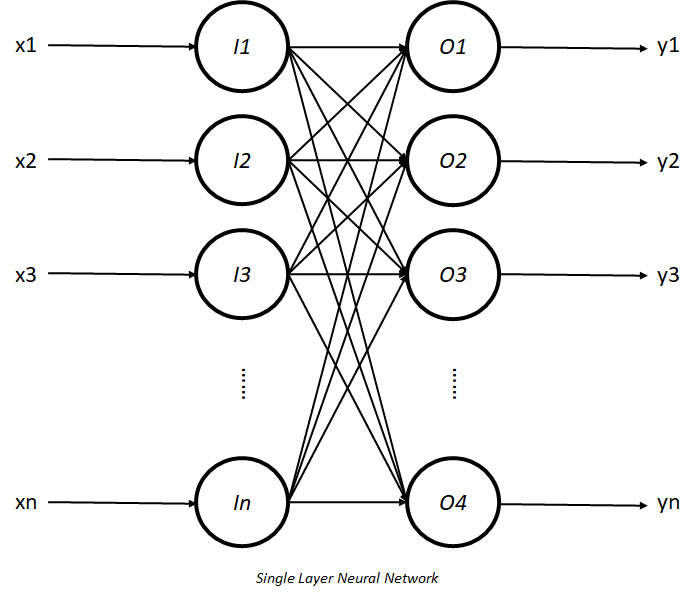Neural networks can grow in complexity by adding additional layers which are commonly referred to as “hidden layers”.  In theory a network can contain an infinite number of layers with an infinite number of neurons although this is neither practical or necessary.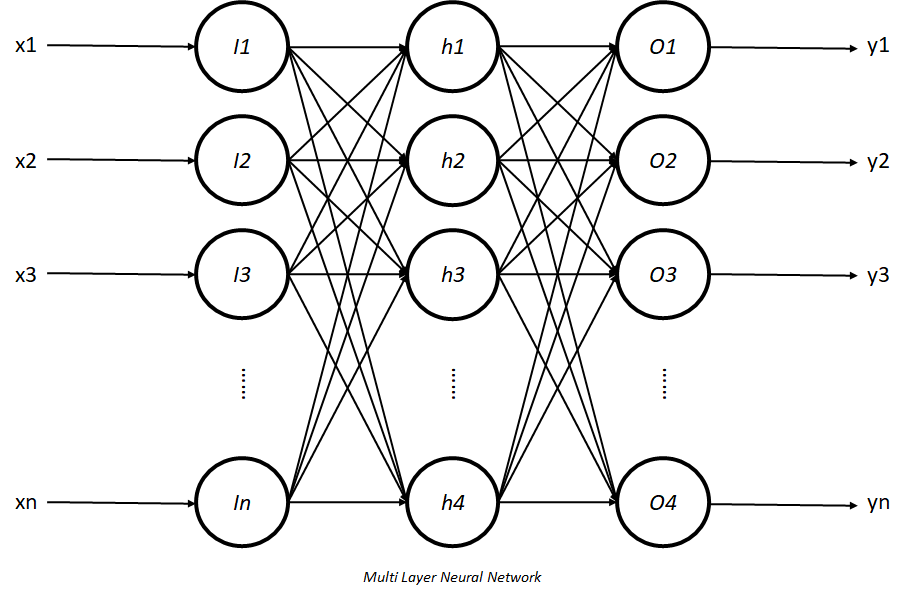The only remaining question then is how do we know what weights will give us the outputs we are looking for.  A simple feed forward neural network uses a technique called forward and back propagation to train the network and find the optimal weights.   There are dozens of books and blog posts devoted to the subject of how the forward and back propagation algorithms work, but for the sake of this blog post I’ll provide an introductory explanation along with pointers to additional information.

The main idea requires randomly initializing our weights and pushing the inputs “forward” through the network so we can make an output prediction.   We then use a cost or loss function to calculate how far our prediction was from the expected result.

Our ultimate goals is to reduce our error or cost to the lowest point possible (sometimes referred to as the global minimum).  To do this we use an algorithm called gradient decent.   The goal of the gradient descent algorithm is to find the partial derivative of the cost function with respect to each weight.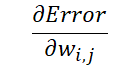In other words we’re looking for the direction (+/-) and slope of our cost function to tell us how large to adjust our weights and in which direction in order to get to zero cost (or close to it).  If the gradient is 0 we have reached our minima.   While I won’t go into the details thanks to the concept of the chain rule in calculus we can actually start at the output layer , perform the gradient descent algorithm, and “back” propagate it to the next layer and all the way back to our inputs.  Along the way we are calculating how much we need to adjust our weights to get closer to that zero cost.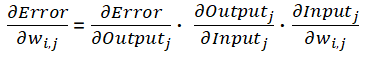When training a neural network we continue to forward and back propagate until we we have minimized the error.  While I have grossly oversimplified the explanation for forward and back propagation, this is fundamentally how neural networks work.  I have provided links to more detailed descriptions at the end of this post.

##### Putting it All Together

Now that we have some basic knowledge of end-to-end speech recognition systems and neural networks, we’re ready to make a simple end-to-end speech recognizer.  To build this recognizer I used python and the numpy library to help with the matrix math.

However, before we start we need a simple speech data set.  Preferably one consisting of utterances with only single words.  This would eliminate the need to deal with time alignment (i.e. which text goes with which audio segment in time).  Luckily I found a great freely available dataset consisting of people speaking single digits 0 – 9 with fifty utterances per digit per person.   This data set met the criteria of being a single word while also being sufficiently large enough to train a neural network.

With labeled audio data in hand the next step required is reading in the audio data and the associated labels  For this I used the python librosa library.  Librosa provides easy to use out-of-the-box functions for computing the Short Time Fourier Transform (STFT) which is necessary to get the frequency spectrum of our audio signal (e.g. our input signal).  Librosa additionally provides handy functions for computing other audio features like Mel Frequency Cepstral Coefficients (MFCC) which can also be a useful audio input feature (note my code provides an alternative implementation that uses MFCC’s instead of the raw spectrum)

```1 2 3 4 5 6 7 8 9 10 11 12 13 14 15 16 for files in file_list: relative_path = 'recordings/' + files file_name = os.path.join(os.path.dirname(__file__), relative_path) y, sr = load(file_name, sr=None) filesize = sys.getsizeof(y)   if output_type == 'spectrum': spectrum = stft(y, nfft, hop_length=int(filesize / 2)) mag, phase = magphase(spectrum) mag_input.append(mag)   mfcc = feature.mfcc(y, sr, n_mfcc=nmfcc, hop_length=int(filesize / 2)) mfcc = mfcc[1:nmfcc] mfcc_input.append(mfcc)   digit.append(files)```

Beyond the audio, we also need to store the associated digit spoken in each audio file.   When training a multiclass classifier ( in our case our classes are 0 – 9) it’s common to use something called “one hot” vectors to represent the output.   This is just a vector where all the classes are represented by 0 except for the one element representing the actual output class.   So in our case we have a 10 element vector and if the audio file is someone saying “one’ the vector would look like [0 1 0 0 0 0 0 0 0 0 ].

```1 2 3 4 5 6 7 8 9 10 11 class digits: zero = [1, 0, 0, 0, 0, 0, 0, 0, 0, 0] one = [0, 1, 0, 0, 0, 0, 0, 0, 0, 0] two = [0, 0, 1, 0, 0, 0, 0, 0, 0, 0] three = [0, 0, 0, 1, 0, 0, 0, 0, 0, 0] four = [0, 0, 0, 0, 1, 0, 0, 0, 0, 0] five = [0, 0, 0, 0, 0, 1, 0, 0, 0, 0] six = [0, 0, 0, 0, 0, 0, 1, 0, 0, 0] seven = [0, 0, 0, 0, 0, 0, 0, 1, 0, 0] eight = [0, 0, 0, 0, 0, 0, 0, 0, 1, 0] nine = [0, 0, 0, 0, 0, 0, 0, 0, 0, 1]```

With our inputs and outputs squared away it’s time to define our network. The variables that make up your network are also known as hyper-parameters. For my end-to-end recognizer I selected the following hyper-parameters: (*Note that selecting hyper-parameters is half art and half science and your choices will be critical to the success of your network.  I have provided additional resources below)

• Number of layers:3 (input, output and one hidden layer)
• Nodes in hidden layer: 2048 (1x our frequency bins)
• Activation functions: TanH (HIdden), Sigmoid (Output)
• Weight initialization algorithm: Xavier or Glorot
• Learning rate = 0.001
• Rate decay = 0.0001
```1 2 3 4 5 6 7 8 9 10 11 12 input_layer = layers.Layer(inputs=training_inputs.shape, neurons=training_inputs.shape + 1)   if mode == 'E2E': hidden_layer = layers.Layer(inputs=training_inputs.shape + 1, neurons=2048, activation=activationfunctions.Tanh_Activation, activation_derivative=activationfunctions.Tanh_Activation_Deriv) hidden_layer.Initialize_Synaptic_Weights()   output_layer = layers.Layer(inputs=2048, neurons=training_outputs.shape, activation=activationfunctions.Sigmoid_Activation, activation_derivative=activationfunctions.Sigmoid_Activation_Derivative) output_layer.Initialize_Synaptic_Weights()if mode == 'E2E':```

nnet = NeuralNetwork(layer1=input_layer, layer2=hidden_layer, layer3=output_layer, learning_rate=0.001,
learning_rate_decay=0.0001, momentum=0.5)

So now that we have our inputs and outputs, and we’ve defined our network, all we need to do is train using our forward and back propagation functions. Per my earlier description the forward propagation algorithm is quite simple and is really just summing the weighted inputs and applying the activation functions. Using matrix math this can be written in three or four simple lines of code.

```1 2 3 4 5 def Feed_Forward(self, inputs): self.l1_inputs[:,0:self.layer1.neurons-1] = inputs self.l2_hidden = self.layer2.activation(dot(self.l1_inputs, self.layer2.synaptic_weights)) self.l3_output = self.layer3.activation(dot(self.l2_hidden, self.layer3.synaptic_weights)) return self.l3_output```

The forward propagation algorithm gives us our predicted output.  Using that predicted output we can perform our back propagation.  Much like my earlier explanation we need to perform a series of steps for each layer.   Specifically we need to calculate the error, calculate the gradient, and adjust our weights based on the previous two calculations.

```1 2 3 4 5 6 7 8 9 10 11 12 13 14 15 16 17 18 19 20 21 22 def Back_Propogate(self, outputs):   output_deltas = numpy.zeros((self.layer1.inputs, self.layer3.neurons)) l3_output_error = -(outputs - self.l3_output) if self.layer3.activation_derivative == activationfunctions.Sigmoid_Activation_Derivative: output_deltas = self.layer3.activation_derivative(self.l3_output) * l3_output_error elif self.layer3.activation_derivative == activationfunctions.softmax_derivative: output_deltas = l3_output_error elif self.layer3.activation_derivative == activationfunctions.Oland_Et_Al_Derivative: output_deltas = self.layer3.activation_derivative(self.l3_output) - outputs   hidden_deltas = numpy.zeros((self.layer1.inputs, self.layer2.neurons)) l2_hidden_error = output_deltas.dot(self.layer3.synaptic_weights.T) hidden_deltas = self.layer2.activation_derivative(self.l2_hidden) * l2_hidden_error   adjustment1 = self.l2_hidden.T.dot(output_deltas) self.layer3.synaptic_weights = self.layer3.synaptic_weights - (adjustment1 * self.learning_rate) #+ self.l3_output_adjustment * self.momentum self.l3_output_adjustment = adjustment1   adjustment2 = self.l1_inputs.T.dot(hidden_deltas) self.layer2.synaptic_weights = self.layer2.synaptic_weights - (adjustment2 * self.learning_rate) #+ self.l2_hidden_adjustment * self.momentum self.l2_hidden_adjustment = adjustment2```

To bring it all together we just need to iterate over our forward and back propagation algorithms until we have stopped learning or have reduced our cost or error to it’s lowest possible point.

```1 2 3 4 5 6 7 8 9 10 11 12 13 14 15 16 17 18 19 def Train(self, inputs, outputs, iterations): for iteration in range(iterations): error = 0.0   # random.shuffle(patterns) # turn off random randomize = numpy.arange(len(inputs)) numpy.random.shuffle(randomize) inputs = inputs[randomize] outputs = outputs[randomize]   self.Feed_Forward(inputs) error = self.Back_Propogate(outputs) error = numpy.average(error) if iteration % 10 == 0: print('error %-.5f' % error) # learning rate decay self.learning_rate = self.learning_rate * ( self.learning_rate / (self.learning_rate + (self.learning_rate * self.learning_rate_decay)))```

That’s it!  While there is a lot more glue code and learning that went into this implementation what I have presented here represents the fundamental building blocks of a basic end-to-end speech recognition system.  I have made the full project available on GitHub and you can evaluate the code yourself in order to fully comprehend all the details.  I’ve also provided a bevy of resources below that helped get me to this point and can do the same for you.

##### Final Thoughts

You might be asking why a senior leader in my position would spend the time required to go through this exercise.  There are some general principles I like to follow and I think anybody managing a research oriented (or really any engineering) team should consider as well.  Specifically:

• ABL – Always Be Learning:  If you want to innovate you need to be up to speed on the latest technology trends.
• Earn your team’s respect:  The best way to earn the respect of your technical team is to get into the trenches.  Show them that you understand their job and all the pain that comes with it.  In other words write code (any code), test it, check it in, and push it to production.
• Lead by example: If you want your team to “innovate for the masses”, it’s best demonstrate the behaviors you are looking for.

Hopefully this post has given you a basic understanding of end-to-end speech recognition systems and neural networks  If you’re really brave perhaps you’ve learned how to build your own simple end-to-end recognizer.  But if you take nothing else away from this article I hope it’s that you’ll invest your time improving your own technical skills and getting in the trenches to earn your team’s respect.

In an upcoming post I’ll dig deeper into end-to-end speech recognition algorithms and how they work.  Specifically we’ll cover recurrent neural networks and the connectionist temporal classification algorithms that truly allow these systems to be superior over traditional speech recognition systems.  In the mean time I hope you get a chance to “wreck a nice beach”!

##### References
1. “How to build a simple neural network in 9 lines of Python code” – Milo Spencer-Harper
2. “How to build a multi-layered neural network in Python” – Milo Spencer-Harper
3. “Understanding and coding Neural Networks from Scratch in Python and R” – Sunil Ray
4. “How to Compute the Derivative of  Sigmoid Function (fully worked example)” – Jeremy (no last name)
5. “Practical recommendation for Gradient-Based Training of Deep Architectures” – Yoshua Bengio
6. “How to train your Deep Neural Network” – Rishabh Shukla
7. “Understanding the difficulty of training deep feedforward neural networks” – Xavier Glorot and Yoshua Bengio
8. “Deep Learning Basics: Neural Networks, Backpropegation, and stochastic Gradient Descent” –  Alex Minnaar
9. “Speech Recognition: You down with CTC” – Karl N.
10. “Deep Speech: Scaling up end-to-end speech recognition” – Andrew Y. Ng et al.
11.  “Connectionist Temporal Classification: Labeling Unsegmented Sequence Data with Recurrent Neural Networks” – Alex Graves et al.